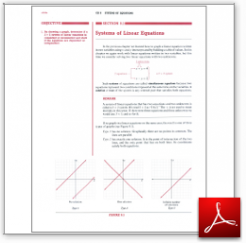Algebra Math Book - Introductory Algebra Thank you for your continual support.

 The power of mathematics rests in the logic of thinking.ID: Sec8-1
Description: Simultaneous Equations
Price: 1.99

# Algebra Math book - Introductory Algebra - Chapter 8 - Section 1 - Systems of Linear Equations

## Section 8.1 - Objectives

1.  By drawing a graph, determine if a 2 x 2 system of linear equations is consistent or inconsistent and state if the equations are dependent or independent.

Navigate to
Next Section
or Chapter 8 Details or Other Chapters

This section of my algebra math book, Introductory Algebra, also includes in the download:

• Cover Sheet